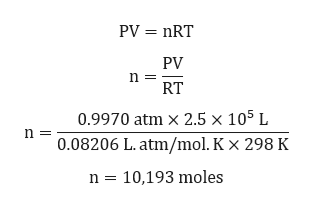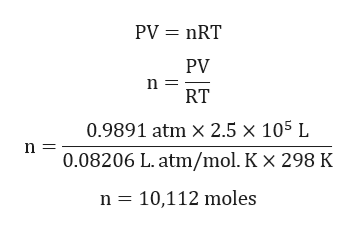# Text prior to questions in "{ }"Another air-purification method for enclosed spaces involves the use of “scrubbers” containing aqueous lithium hydroxide, which react with carbon dioxide to produce lithium carbonate and water.2LiOH(aq) + CO2(g)  Li2CO3(s) + H2O(l)Consider the air supply in a submarine with a total volume of 2.5 × 105 L. The pressure is 0.9970 atm, and the temperature is 25°C. If the pressure in the submarine drops to 0.9891 atm as the result of carbon dioxide being consumed by an aqueous lithium hydroxide scrubber, how many moles of CO2 are consumed?Strategy Use Equation 11.19(b) to determine Δn, the number of moles of CO2 consumed.Setup ΔP = 0.9970 atm − 0.9891 atm = 7.9 × 10−3 atm. According to the problem statement, V = 2.5 × 105 L and T = 298.15 K. For problems in which P is expressed in atmospheres and V in liters, use R = 0.08206 L · atm/K · mol.Solution Think About ItCareful cancellation of units is essential. Note that this amount of CO2 corresponds to 162 moles or 3.9 kg of LiOH. (It’s a good idea to verify this yourself.)]Practice Problem UILD  By how much would the pressure in the submarine drop if 2.55 kg of LiOH were completely consumed by reaction with CO2? (Assume the same starting P, V, and T as in Worked Example 11.15.)

Question
36 views

Text prior to questions in "{ }"

Another air-purification method for enclosed spaces involves the use of “scrubbers” containing aqueous lithium hydroxide, which react with carbon dioxide to produce lithium carbonate and water.

2LiOH(aq) + CO2(g)  Li2CO3(s) + H2O(l)

Consider the air supply in a submarine with a total volume of 2.5 × 105 L. The pressure is 0.9970 atm, and the temperature is 25°C. If the pressure in the submarine drops to 0.9891 atm as the result of carbon dioxide being consumed by an aqueous lithium hydroxide scrubber, how many moles of CO2 are consumed?

Strategy Use Equation 11.19(b) to determine Δn, the number of moles of CO2 consumed.

Setup ΔP = 0.9970 atm − 0.9891 atm = 7.9 × 10−3 atm. According to the problem statement, V = 2.5 × 105 L and T = 298.15 K. For problems in which P is expressed in atmospheres and V in liters, use R = 0.08206 L · atm/K · mol.

Solution

Careful cancellation of units is essential. Note that this amount of CO2 corresponds to 162 moles or 3.9 kg of LiOH. (It’s a good idea to verify this yourself.)]

Practice Problem UILD  By how much would the pressure in the submarine drop if 2.55 kg of LiOH were completely consumed by reaction with CO2? (Assume the same starting P, V, and T as in Worked Example 11.15.)

check_circle

Step 1

Given chemical reaction:

2LiOH(aq) + CO2(g) → Li2CO3(s) + H2O(l)

Step 2

Calculation for initial number of moles:help_outlineImage TranscriptionclosePV = nRT PV RT 0.9970 atm x 2.5 × 105 L 0.08206 L. atm/mol. K x 298 K n = 10,193 moles fullscreen
Step 3

Calculation for final n...help_outlineImage TranscriptionclosePV = nRT PV n RT 0.9891 atm × 2.5 × 105 L n 0.08206 L. atm/mol. K x 298 K n = 10,112 moles fullscreen

### Want to see the full answer?

See Solution

#### Want to see this answer and more?

Solutions are written by subject experts who are available 24/7. Questions are typically answered within 1 hour.*

See Solution
*Response times may vary by subject and question.
Tagged in

### Chemistry